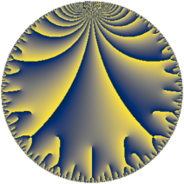Properties

 Label 98.10.cLevel $98$ Weight $10$ Character orbit 98.c Rep. character $\chi_{98}(67,\cdot)$ Character field $\Q(\zeta_{3})$ Dimension $60$ Newform subspaces $14$ Sturm bound $140$ Trace bound $3$

Related objects

Defining parameters

 Level: $$N$$ $$=$$ $$98 = 2 \cdot 7^{2}$$ Weight: $$k$$ $$=$$ $$10$$ Character orbit: $$[\chi]$$ $$=$$ 98.c (of order $$3$$ and degree $$2$$) Character conductor: $$\operatorname{cond}(\chi)$$ $$=$$ $$7$$ Character field: $$\Q(\zeta_{3})$$ Newform subspaces: $$14$$ Sturm bound: $$140$$ Trace bound: $$3$$ Distinguishing $$T_p$$: $$3$$

Dimensions

The following table gives the dimensions of various subspaces of $$M_{10}(98, [\chi])$$.

Total New Old
Modular forms 268 60 208
Cusp forms 236 60 176
Eisenstein series 32 0 32

Trace form

 $$60 q + 162 q^{3} - 7680 q^{4} + 1818 q^{5} - 9728 q^{6} - 210336 q^{9} + O(q^{10})$$ $$60 q + 162 q^{3} - 7680 q^{4} + 1818 q^{5} - 9728 q^{6} - 210336 q^{9} - 5632 q^{10} - 40718 q^{11} + 41472 q^{12} - 233176 q^{13} - 267380 q^{15} - 1966080 q^{16} + 285906 q^{17} - 42944 q^{18} - 842658 q^{19} - 930816 q^{20} + 833920 q^{22} + 6297866 q^{23} + 1245184 q^{24} - 16007928 q^{25} + 1287168 q^{26} - 27125676 q^{27} + 29525768 q^{29} + 15638400 q^{30} + 16032506 q^{31} + 7030126 q^{33} - 10477568 q^{34} + 107692032 q^{36} + 19039542 q^{37} + 25578240 q^{38} - 38593300 q^{39} - 1441792 q^{40} - 68514696 q^{41} - 110530232 q^{43} - 10423808 q^{44} + 123651196 q^{45} - 1829504 q^{46} + 4311750 q^{47} - 21233664 q^{48} + 186286592 q^{50} - 22812154 q^{51} + 29846528 q^{52} + 30006982 q^{53} + 124568320 q^{54} + 79122004 q^{55} + 907882428 q^{57} + 166721280 q^{58} - 131270238 q^{59} + 34224640 q^{60} - 120684718 q^{61} + 87151104 q^{62} + 1006632960 q^{64} - 196155036 q^{65} - 232417280 q^{66} - 325396866 q^{67} + 73191936 q^{68} + 676075180 q^{69} - 936287376 q^{71} - 10993664 q^{72} - 865220822 q^{73} - 683193088 q^{74} - 918577000 q^{75} + 431440896 q^{76} - 1292151296 q^{78} - 1235771846 q^{79} + 119144448 q^{80} + 197249438 q^{81} + 541421568 q^{82} - 146819472 q^{83} + 6047298844 q^{85} - 1663555392 q^{86} - 2165628028 q^{87} - 106741760 q^{88} + 95513418 q^{89} - 1566402560 q^{90} - 3224507392 q^{92} + 2582500138 q^{93} + 1617508608 q^{94} - 1216392650 q^{95} + 318767104 q^{96} + 3896128312 q^{97} + 11536386544 q^{99} + O(q^{100})$$

Decomposition of $$S_{10}^{\mathrm{new}}(98, [\chi])$$ into newform subspaces

Label Dim. $$A$$ Field CM Traces $q$-expansion
$a_{2}$ $a_{3}$ $a_{5}$ $a_{7}$
98.10.c.a $2$ $50.474$ $$\Q(\sqrt{-3})$$ None $$-16$$ $$-170$$ $$-544$$ $$0$$ $$q-2^{4}\zeta_{6}q^{2}+(-170+170\zeta_{6})q^{3}+\cdots$$
98.10.c.b $2$ $50.474$ $$\Q(\sqrt{-3})$$ None $$-16$$ $$-156$$ $$870$$ $$0$$ $$q-2^{4}\zeta_{6}q^{2}+(-156+156\zeta_{6})q^{3}+\cdots$$
98.10.c.c $2$ $50.474$ $$\Q(\sqrt{-3})$$ None $$-16$$ $$156$$ $$-870$$ $$0$$ $$q-2^{4}\zeta_{6}q^{2}+(156-156\zeta_{6})q^{3}+(-2^{8}+\cdots)q^{4}+\cdots$$
98.10.c.d $2$ $50.474$ $$\Q(\sqrt{-3})$$ None $$-16$$ $$170$$ $$544$$ $$0$$ $$q-2^{4}\zeta_{6}q^{2}+(170-170\zeta_{6})q^{3}+(-2^{8}+\cdots)q^{4}+\cdots$$
98.10.c.e $2$ $50.474$ $$\Q(\sqrt{-3})$$ None $$16$$ $$-6$$ $$560$$ $$0$$ $$q+2^{4}\zeta_{6}q^{2}+(-6+6\zeta_{6})q^{3}+(-2^{8}+\cdots)q^{4}+\cdots$$
98.10.c.f $2$ $50.474$ $$\Q(\sqrt{-3})$$ None $$16$$ $$6$$ $$-560$$ $$0$$ $$q+2^{4}\zeta_{6}q^{2}+(6-6\zeta_{6})q^{3}+(-2^{8}+2^{8}\zeta_{6})q^{4}+\cdots$$
98.10.c.g $4$ $50.474$ $$\Q(\sqrt{-3}, \sqrt{106})$$ None $$-32$$ $$0$$ $$0$$ $$0$$ $$q+2^{4}\beta _{2}q^{2}+3\beta _{1}q^{3}+(-2^{8}-2^{8}\beta _{2}+\cdots)q^{4}+\cdots$$
98.10.c.h $4$ $50.474$ $$\Q(\sqrt{-3}, \sqrt{2305})$$ None $$32$$ $$-14$$ $$-2730$$ $$0$$ $$q+(2^{4}-2^{4}\beta _{1})q^{2}+(-7\beta _{1}-5\beta _{2})q^{3}+\cdots$$
98.10.c.i $4$ $50.474$ $$\Q(\sqrt{-3}, \sqrt{43})$$ None $$32$$ $$0$$ $$0$$ $$0$$ $$q-2^{4}\beta _{2}q^{2}+\beta _{1}q^{3}+(-2^{8}-2^{8}\beta _{2}+\cdots)q^{4}+\cdots$$
98.10.c.j $4$ $50.474$ $$\Q(\sqrt{-3}, \sqrt{2305})$$ None $$32$$ $$14$$ $$2730$$ $$0$$ $$q+(2^{4}-2^{4}\beta _{1})q^{2}+(7\beta _{1}+5\beta _{2})q^{3}+\cdots$$
98.10.c.k $6$ $50.474$ $$\mathbb{Q}[x]/(x^{6} - \cdots)$$ None $$-48$$ $$233$$ $$733$$ $$0$$ $$q+(-2^{4}+2^{4}\beta _{2})q^{2}+(78\beta _{2}-\beta _{4})q^{3}+\cdots$$
98.10.c.l $6$ $50.474$ $$\mathbb{Q}[x]/(x^{6} - \cdots)$$ None $$48$$ $$-71$$ $$1085$$ $$0$$ $$q+2^{4}\beta _{1}q^{2}+(-24+24\beta _{1}-\beta _{2}+\beta _{4}+\cdots)q^{3}+\cdots$$
98.10.c.m $8$ $50.474$ $$\mathbb{Q}[x]/(x^{8} - \cdots)$$ None $$64$$ $$0$$ $$0$$ $$0$$ $$q+(2^{4}-2^{4}\beta _{1})q^{2}+(-\beta _{2}+5\beta _{3})q^{3}+\cdots$$
98.10.c.n $12$ $50.474$ $$\mathbb{Q}[x]/(x^{12} - \cdots)$$ None $$-96$$ $$0$$ $$0$$ $$0$$ $$q-2^{4}\beta _{1}q^{2}+(\beta _{8}+\beta _{10})q^{3}+(-2^{8}+\cdots)q^{4}+\cdots$$

Decomposition of $$S_{10}^{\mathrm{old}}(98, [\chi])$$ into lower level spaces

$$S_{10}^{\mathrm{old}}(98, [\chi]) \cong$$ $$S_{10}^{\mathrm{new}}(7, [\chi])$$$$^{\oplus 4}$$$$\oplus$$$$S_{10}^{\mathrm{new}}(14, [\chi])$$$$^{\oplus 2}$$$$\oplus$$$$S_{10}^{\mathrm{new}}(49, [\chi])$$$$^{\oplus 2}$$